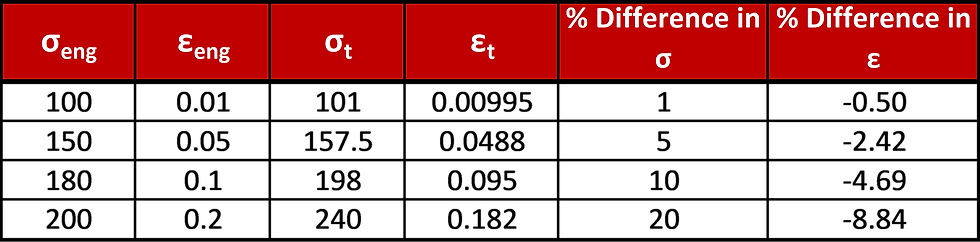top of page
Search

# True-Stress And True-Strain – What Is It And Why Does It Matter?

It is likely that most simulation engineers have at least heard of the terms true-stress and true-strain before. But what do these terms mean, how do we calculate them and why are they important? This post aims to address all three of these questions.

## Engineering-Stress and Strain

Before we delve into true-stress and strain, it is probably important that we understand what comes from the traditional tensile testing that we do on many of our materials in the laboratory. A standard tensile test produces what we typically refer to as engineering or nominal stress and strain. Essentially, the sample in question is stretched using a testing machine and the displacements caused by the applied load are measured and recorded.Of course, given that we know the initial shape of the specimen, we can infer the level of stressand strainat any point during the test, meaning we can simply convert our unitless load-displacement plot into an ‘engineering’ stress-strain plot.The problem with this approach to generate stress-strain data is not obvious at first, but with a little consideration, it becomes apparent. While engineering stress and strain inferred directly from force-displacement data might be reasonable at the beginning of loading, the specimen is changing shape as load is increased, meaning that the values that we used in our calculations above, namely original length and specimen cross-section, become less and less valid or true. The specimen is thinning due to the Poisson’s ratio and is also increasing in length, meaning that stress is actually higher than in our nominal calculation and incremental strain is lower.## True-Stress and Strain

So now we know why the engineering strain isn’t exactly right, what is? Well, the answer is true-stress and strain. So, what are these and how do we calculate them?

Under the assumption that the volume of material remains constant throughout the test, taking the product of the cross-section and the gauge length yields the same result, regardless of what stage of the test this is done at. This obviously only holds true prior to necking of the specimen, at which point all bets are off and the stress at failure must be calculated after the fact by measuring the area of the fracture surface.

Hence:OrWhere subscript o denotes original and subscript i denotes instantaneous.

If we know that true-stress is force over instantaneous area and that engineering strain is change in length over original length, then we can manipulate the equations above to allow calculation of true-stress from only engineering stress and strain as shown here:Now, the true-strain is simply the change in instantaneous length over time, which can be expressed as an integral:And remembering that engineering strain can be expressed as:Then we can replace length in the true-strain equation with engineering strain as follows:## Does It Matter?

As mentioned previously, there is not much disparity between engineering and true stress and strain values at low strain. However, as loading increases and the specimen begins to change shape more drastically, the difference does become relevant and, hence, we would always recommend utilizing true-stress and strain data in your FEA models.

Let’s take a look at the error in the stress-strain data for an arbitrary material at various strain levels:As we can see presented in the data above, once the strain in the model increases beyond around 5%, the conversion to true-stress true-strain certainly does begin to matter. In fact, the error in stress is directly related to the engineering strain and the difference between the stress in the curves at 18.2% strain is a whopping 22.5%.

## Final Thoughts

True-stress and strain are probably at least in the vocabulary of most structural simulation engineers. Hopefully this post has shed some light on what exactly these values are and why you should probably be using them in your FEA models, especially when the amount of strain in the model exceeds a couple of percent.

As always, we’re here to help! Get in touch with our team today to learn more about Fidelis what we can offer you!

3,674 views
bottom of page